# Export and Import Excel Data in C#

## Export and Import Excel Data in C# using the NPOI Library

In this article, I am going to discuss How to Export and Import Excel Data in C# with Examples using the NPOI Library. Please read our previous article, where we discussed the DirectoryInfo Class in C# with Examples. Whenever we are working on Real-Time Projects, it is a common requirement to Import data from an Excel File as well as to Export Data to an Excel File. So, here, first, we will discuss how to Export Excel Data, and then we will see How to Import Excel Data using NPOI Library.

##### What is NPOI?

NPOI is an Open-Source library that can help us perform read and write operations on XLS, DOC, and PPT file extensions. This NPOI Library is the .NET version of the POI Java project at http://poi.apache.org/. This NPOI Library covers almost all the important features of Excel, like styling, formatting, data formulas, extracting images, etc. And the most important thing is that it does not require Microsoft Office to be installed on the server. So, here, in this article, I will show you how to perform the Read and Write Operations on an Excel File using the NPOI Library.

##### Example to Understand Export Excel Data in C#:

Let us see an Example to Understand How to Export Excel Data in C# using the NPOI Library. So, we want to represent the Employee information of an organization in the following Excel Format.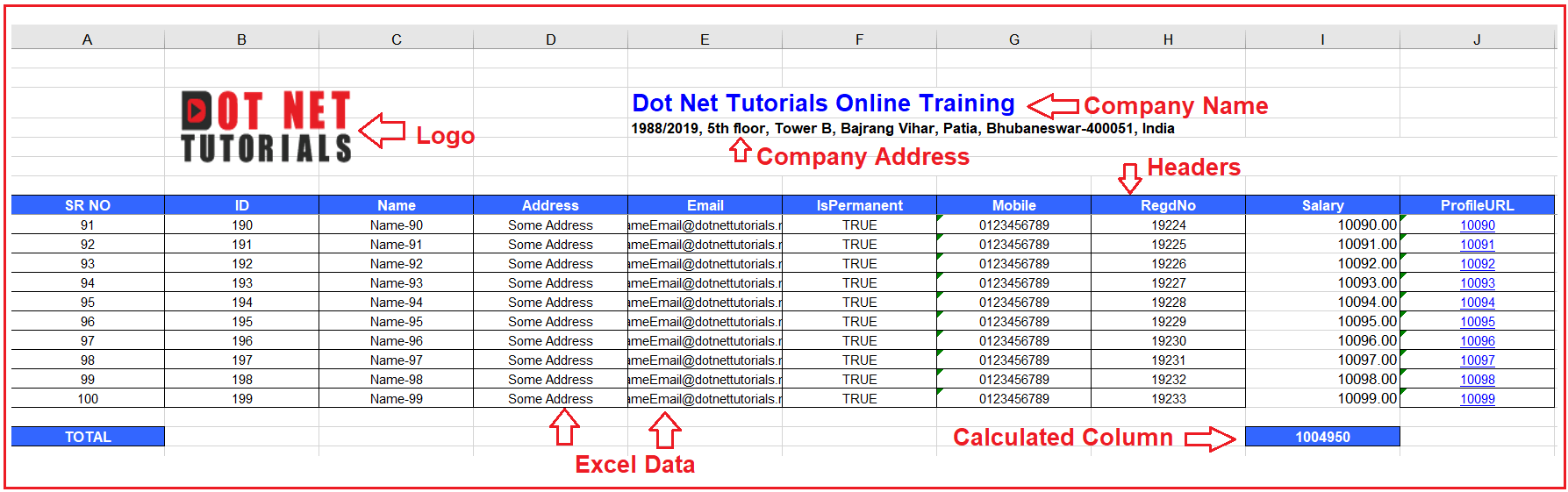As you can see in the above image, our Excel File is going to contain the following information.

On the Left-Hand Side top corner, we want to put the logo of the Company. On the right-hand side, we want to put the Company Name and Company Address. Here, we need to apply different Cell Styles for Company Name and Address.

Then, we need to provide the column headers, and for this, we are going to use different Cell Styles. After the Column Header, we have Data Rows, and for Data Rows, we have different cell styles. We have a different cell style for string data and another cell style for numeric data. Further, if you observe the ProfileURL column, we need to make that column value a Hyperlink, and on clicking on that hyperlink, it should open the appropriate URL on your browser. For this hyperlink column data, we have a different cell style.

Once the Data Rows are completed, we need to calculate the total salary. For this, we need to use Excel Formula, and for this, we are using a different cell style. The formula will be to sum all the column values of the Salary column and show the result on the Total Row.

Here, we also need to freeze the company logo, company name, company address, and headers while scrolling down to see the data rows. Let us proceed and see how to implement the above-discussed example in C# using the NPOI Library.

##### Export Excel Data Example in C# using NPOI Library:

So, create a Console application with the name WorkingWithExcel. Once you create the Project, then you need to install the NPOI Library from NuGet Package Manager. To do so, Click on Tools => NuGet Package Manager => Manage NuGet Packages for Solution, as shown in the below image.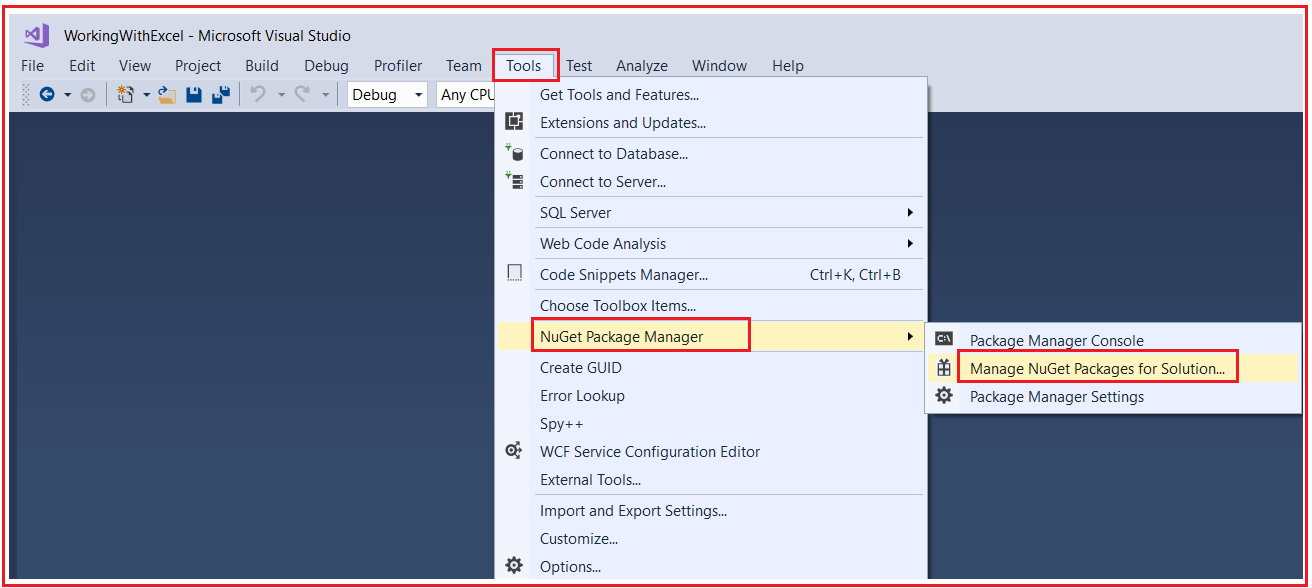Then go to the Browse tab, search for NPOI, and select the NPOI.Excel Library, select the project where you want to install the Library, and finally click on the Install button as shown in the below image.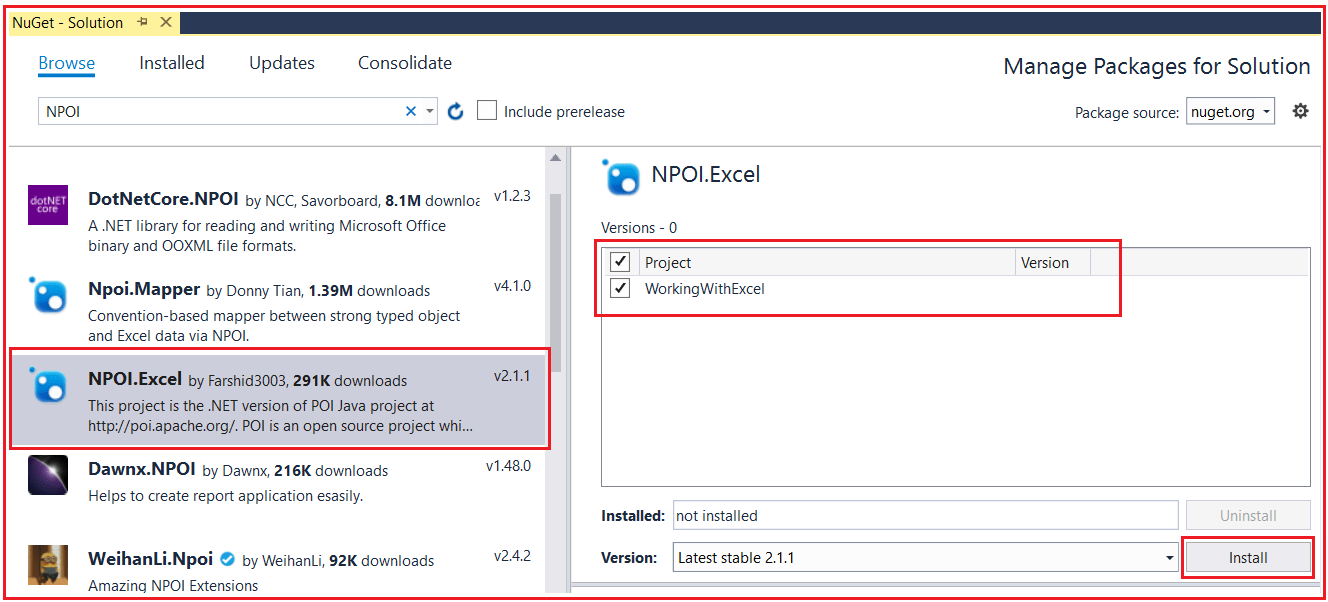Once you have Installed the NPOI Library, create a folder with the name Images, and inside this folder, please download and save the following image.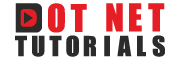Next, create another folder with the name ExcelFiles and inside this folder, our generated Excel Files will be stored. Next, create a class file with the name Employee.cs and then copy and paste the following code. This is going to be our model class, which is going to hold the Employee data that we want to export into the Excel File.

```namespace WorkingWithExcel
{
public class Employee
{
public int ID { get; set; }
public string Name { get; set; }
public string Address { get; set; }
public string Email { get; set; }
public string Mobile { get; set; }
public bool IsPermanent { get; set; }
public int RegdNo { get; set; }
public int Salary { get; set; }
public string ProfileURL { get; set; }
}
}
```

Next, modify the Program class as follows. In the code below, we use the NPOI Library to Export the Data into an Excel file. The following Example code is self-explained, so please go through the comment lines for a better understanding.

```using System;
using System.Collections.Generic;
using NPOI.HSSF.UserModel;
using NPOI.HSSF.Util;
using NPOI.SS.Util;
using NPOI.SS.UserModel;
using System.IO;

namespace WorkingWithExcel
{
public class Program
{
public static void Main()
{
try
{
#region Data
//In Real-Time you will get the data from the database,
//but here we have hard-coded the data
List<Employee> empList = new List<Employee>();

for (int i = 0; i < 100; i++)
{
Employee emp = new Employee()
{
ID = 100 + i,
Name = "Name-" + i,
Email = "SameEmail@dotnettutorials.net",
IsPermanent = true,
Mobile = "0123456789",
RegdNo = 12345 + i + 6789,
Salary = 10000 + i,
ProfileURL = "100" + i
};
}
#endregion

#region Creating Excel Sheet
//The following Pieces of Code will Create the Excel File,
//Excel Sheet and font object which will be used later
HSSFWorkbook workbook = new HSSFWorkbook();
//The sheet name is going to be Dot Net Tutorials
HSSFSheet sheet = (HSSFSheet)workbook.CreateSheet("Dot Net Tutorials");
HSSFFont font = (HSSFFont)workbook.CreateFont();
#endregion

#region Creating Different Cell Styles
//Now, we going to create different cell styles for different data

#region Company Cell Styles
//We will use the following cell style with the Company Name
var Company = workbook.CreateCellStyle();
Company.Alignment = HorizontalAlignment.Left;
var CompanyFont = workbook.CreateFont();
CompanyFont.FontName = "Arial";
CompanyFont.Color = HSSFColor.Blue.Index;
CompanyFont.Boldweight = (short)FontBoldWeight.Bold;
CompanyFont.FontHeightInPoints = ((short)16);
Company.SetFont(CompanyFont);
#endregion

//We will use the following cell style with the Company Address
#endregion

//We will use the following cell style with the Header
#endregion

#region Number Data Cell Style
//We will use the following cell style with Data which is Numeric
var NumData = workbook.CreateCellStyle();
var formatId = HSSFDataFormat.GetBuiltinFormat("##0.00");
if (formatId == -1)
{
var newDataFormat = workbook.CreateDataFormat();
NumData.DataFormat = newDataFormat.GetFormat("##0.00");
}
else
NumData.DataFormat = formatId;
#endregion

#region Data Cell Style
//We will use the following cell style with Data that is NOT Numeric
var Data = workbook.CreateCellStyle();
Data.Alignment = HorizontalAlignment.Center;

var DataFont = workbook.CreateFont();
DataFont.FontName = "Arial";
DataFont.FontHeightInPoints = ((short)9);
Data.SetFont(DataFont);
Data.BorderLeft = BorderStyle.Thin;
Data.BorderTop = BorderStyle.Thin;
Data.BorderRight = BorderStyle.Thin;
Data.BorderBottom = BorderStyle.Thin;
#endregion

//We will use the following cell style with Hyperlink Data

#endregion

#endregion

#region Creating Company and Address of the Excel Sheet
//Creating the Company Name from 2nd Row by applying Company Cell Style
// rowIndex is going to hold the Row Number. Inex means 0 - Based Index
int rowIndex = 2; //rowIndex 2 means 3rd Row
var row = sheet.CreateRow(rowIndex);
var cell = row.CreateCell(4);
cell.SetCellValue("Dot Net Tutorials Online Training");
cell.CellStyle = Company;

//Creating the Company Address from 3rd Row by applying Address Cell Style
rowIndex = rowIndex + 1;
var row1 = sheet.CreateRow(rowIndex);
var cell1 = row1.CreateCell(4);
cell1.SetCellValue("1988/2019, 5th floor, Tower B, Bajrang Vihar, Patia, Bhubaneswar-400051, India");

#endregion

// Set Row index for Header
rowIndex = 7; //rowIndex 7 means 8th Row which is going to be our Header in Excel Sheet
var SR_NO = 0; //We want a unique Serial Number for Each Row in the Excel Sheet

#endregion

#region Excel Data
foreach (Employee data in empList)
{
//Increase the rowIndex and SR_NO by 1 for each record in the empList
rowIndex = rowIndex + 1; //This will be the row number in the Excel Sheet
SR_NO = SR_NO + 1; //Unique Serial Number
var cellindex = 0; //Cell Number starting from 0

//Create the New Row
var gridrow = sheet.CreateRow(rowIndex);
//Create the first Cell in the new Row
var gridcell = gridrow.CreateCell(cellindex);
gridcell.SetCellValue(SR_NO);
//Apply appropriate CSS Styles
gridcell.CellStyle = Data;

//Increse the Cell Index by 1 to create the next cell in the Row
cellindex = cellindex + 1;
//Create the new cell
gridcell = gridrow.CreateCell(cellindex);
gridcell.SetCellValue(data.ID);
//Apply appropriate CSS Styles
gridcell.CellStyle = Data;

//The Process will continue till the last cell in the Row

cellindex = cellindex + 1;
gridcell = gridrow.CreateCell(cellindex);
gridcell.SetCellValue(data.Name);
gridcell.CellStyle = Data;

cellindex = cellindex + 1;
gridcell = gridrow.CreateCell(cellindex);
gridcell.CellStyle = Data;

cellindex = cellindex + 1;
gridcell = gridrow.CreateCell(cellindex);
gridcell.SetCellValue(data.Email);
gridcell.CellStyle = Data;

cellindex = cellindex + 1;
gridcell = gridrow.CreateCell(cellindex);
gridcell.SetCellValue(data.IsPermanent);
gridcell.CellStyle = Data;

cellindex = cellindex + 1;
gridcell = gridrow.CreateCell(cellindex);
gridcell.SetCellValue(data.Mobile);
gridcell.CellStyle = Data;

cellindex = cellindex + 1;
gridcell = gridrow.CreateCell(cellindex);
gridcell.SetCellValue(data.RegdNo);
gridcell.CellStyle = Data;

cellindex = cellindex + 1;
gridcell = gridrow.CreateCell(cellindex);
gridcell.SetCellValue(Convert.ToDouble(data.Salary));
gridcell.CellStyle = NumData;

cellindex = cellindex + 1;
gridcell = gridrow.CreateCell(cellindex);
gridcell.SetCellValue(data.ProfileURL);
//Setting the Cell value as Hyper link
{
//On click on the Hyperlink, it will open the following URL in the browser
};
}
#endregion

#region Freezing Point
//Setting the Freezing Point- Column 0 and Row Number 8
sheet.CreateFreezePane(0, 8, 0, 8);
for (int i = 0; i <= cellheaderindex; i++)
{
sheet.SetColumnWidth(i, 5000);
}
#endregion

#region TOTAL & FORMALA SECTION
//We need to calculate the sum of the salary column
var startrow = 9; //Data Rows started from Row Number 9
var lastdatarow = rowIndex + 1; //Last Row
rowIndex = rowIndex + 2; //Creating a New Rows to display the Total
var Formularow = sheet.CreateRow(rowIndex);
var Formulacell = Formularow.CreateCell(0);
Formulacell.SetCellValue("TOTAL");

//Creating a Cell to display the Total
Formulacell = Formularow.CreateCell(8);
//Formula to calculate the Total
String strFormula = "SUBTOTAL(9,I" + Convert.ToString(startrow) + ":I" + Convert.ToString(lastdatarow) + ")";
Formulacell.SetCellType(CellType.Formula);
Formulacell.SetCellFormula(strFormula);
HSSFFormulaEvaluator.EvaluateAllFormulaCells(workbook);

#endregion

#region LOGO
//Displaying the Logo on the TOP Left Corner of the Excel Sheet
HSSFPatriarch patriarch = (HSSFPatriarch)sheet.CreateDrawingPatriarch();
HSSFClientAnchor anchor = new HSSFClientAnchor(0, 0, 0, 255, 1, 2, 2, 5)
{
AnchorType = (int)NPOI.SS.UserModel.AnchorType.MoveAndResize
};
//Here, you need to replace the Image Path and Name as per your directory structure and Image Name
HSSFPicture picture = (HSSFPicture)patriarch.CreatePicture(anchor, LoadImage(@"C:\Users\HP\source\repos\WorkingWithExcel\WorkingWithExcel\Images\DotNetTutorials.png", workbook));
picture.Resize();
picture.LineStyle = (LineStyle)HSSFPicture.LINESTYLE_NONE;

#endregion

//Finally, Create the Excel File and Save it on a specified Location
string FileName = "MyExcel_" + DateTime.Now.ToString("yyyy-dd-MM--HH-mm-ss") + ".xls";
//Here, you need to replace the Path as per your directory structure where you want to save the image
using (FileStream file = new FileStream(@"C:\Users\HP\source\repos\WorkingWithExcel\WorkingWithExcel\ExcelFiles\" + FileName, FileMode.Create))
{
workbook.Write(file);
file.Close();
Console.WriteLine("File Created Successfully...");
}
}
catch (Exception ex)
{
Console.WriteLine(ex.Message);
}
}
//The following code is used to load the Image, Create the Image and return that image
public static int LoadImage(string path, HSSFWorkbook wb)
{
FileStream file = new FileStream(path, FileMode.Open, FileAccess.Read);
byte[] buffer = new byte[file.Length];
}
}
}

```

With the above changes in place, now run the application code and see the output. If everything is fine then you will get the message saying File Created Successfully…. Now, go to the Excel Files folder and you will see the Excel should be generated with the required format, formula, and data.

Note: Styling the Header, Data, etc. are optional. If you don’t want to, you can remove them.

##### Import Excel Sheet Data in C# using NPOI Library:

Now, let us proceed and understand how to Import Excel Data in C# using the NPOI Library. Let us assume we have the following MyExcelFile.xlsx file in our Excel Files folder.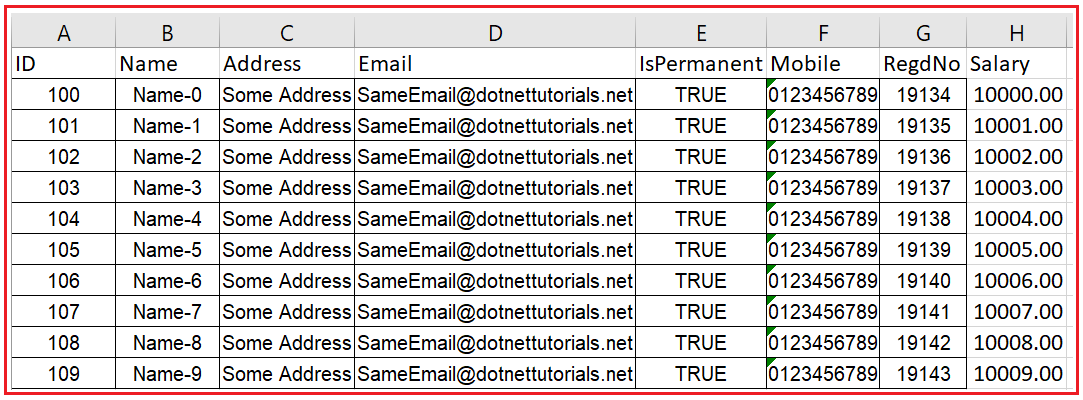As you can see in the above image, this is nothing but the employee data of an organization. Now, we need to import the above Excel Sheet into our .NET Application. So, for this, please modify the Program class as follows. The following code is self-explained, so please go through the comment lines for a better understanding.

```using System;
using System.Collections.Generic;
using NPOI.SS.UserModel;
using System.IO;
using NPOI.XSSF.UserModel;
namespace WorkingWithExcel
{
public class Program
{
public static void Main()
{
try
{
//The following Collection object is going to hold the Excel Sheet Data
List<Employee> listEmployees = new List<Employee>();

//Set the Excel File Path
string FilePath = @"C:\Users\HP\source\repos\WorkingWithExcel\WorkingWithExcel\ExcelFiles\MyExcelFile.xlsx";

//For Import we need to create an instance of XSSFWorkbook class
XSSFWorkbook xssfWorkbook;
using (FileStream file = new FileStream(FilePath, FileMode.Open, FileAccess.Read))
{
xssfWorkbook = new XSSFWorkbook(file);
}

//The Excel File Might have multiple sheets
//So create an ISheet object by specifying the appropriate sheet name or index position
//ISheet sheet = xssfWorkbook.GetSheet("Sheet1"); //Based on the Sheet Name
ISheet sheet = xssfWorkbook.GetSheetAt(0); //Based on the Index Position (0-Based Index Position)

//If First Row is Header, then Initialize the row = 1 else row = 0 inside the for loop
//As our Excel Sheet Contains the First Row as a Header, we are setting row = 1
//The following Loop will start from the First data Rows till the last data row in the sheet
for (int row = 1; row <= sheet.LastRowNum; row++)
{
//null is when the row only contains empty cells
if (sheet.GetRow(row) != null)
{
//If the Row is not empty, then Fetch cell value and populate them with Employee Object
Employee emp = new Employee()
{
//For Accessing Numeric Cell Value we need to use NumericCellValue Property
//For Accessing String Cell Value we need to use StringCellValue Property
//For Accessing Boolean Cell Value we need to use BooleanCellValue Property

//First Cell is ID
ID = (int)sheet.GetRow(row).GetCell(0).NumericCellValue,
//Second Cell is Name
Name = sheet.GetRow(row).GetCell(1).StringCellValue,
//Fourth Cell is Email
Email = sheet.GetRow(row).GetCell(3).StringCellValue,

//Fifth Cell is IsPermanent
IsPermanent = sheet.GetRow(row).GetCell(4).BooleanCellValue,
//Sixth Cell is Mobile
Mobile = sheet.GetRow(row).GetCell(5).StringCellValue,
//Seventh Cell is RegdNo
RegdNo = (int)sheet.GetRow(row).GetCell(6).NumericCellValue,
//Eighth Cell is Salary
Salary = (int)sheet.GetRow(row).GetCell(7).NumericCellValue,
};

//Add the employee data into listEmployees Collection
}
}

//Once you import the data from the Excel File to your .NET Object, then you can do whatever you want
//Like Save the Data into the Database, Display the Data, Modify the Data, etc
//Here, we are going to Display the Excel Sheet Data
foreach (var emp in listEmployees)
{
}
}
catch (Exception ex)
{
Console.WriteLine(ex.Message);
}
}
}

public class Employee
{
public int ID { get; set; }
public string Name { get; set; }
public string Address { get; set; }
public string Email { get; set; }
public string Mobile { get; set; }
public bool IsPermanent { get; set; }
public int RegdNo { get; set; }
public int Salary { get; set; }
}
}
```
###### Output: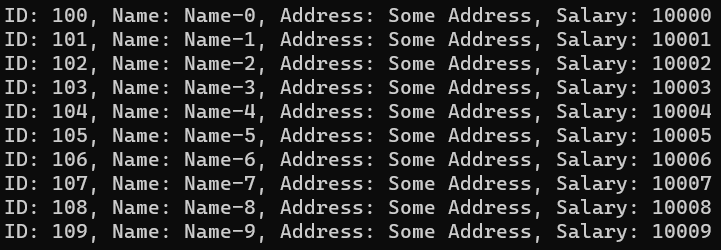In the next article, I will discuss How to generate PDF in C# with Examples. Here, in this article, I try to explain How to Perform Export and Import Excel Data in C# with Examples using the NPOI Library. I hope you enjoy this Export and Import Excel Data in C# with Examples article.